# Functional-differential equation

(diff) ← Older revision | Latest revision (diff) | Newer revision → (diff)

Many real systems exhibit the phenomenon of after-effect, which means that future states of the system can depend not only on the present, but also on the past history. After-effect occurs in physics, chemistry, biology, economy, medicine, cybernetics, etc. This wide appearance of after-effect is a reason to consider it as a universal property of the surrounding world. An appropriate mathematical tool to investigate phenomena with after-effect is provided by the theory of functional-differential equations. Historically, the first functional-differential equations were considered by L. Euler, J. Bernoulli, J. Lagrange, P. Laplace, S.D. Poisson and others in the 18th century in connection with geometrical problems. In the 19th century, functional-differential equations were investigated rather occasionally. At the beginning of the 20th century, important applications of functional-differential equations in the mechanics of visco-elasticity and in ecology were found by V. Volterra [a5], [a6]. The situation changed radically in the 1930s due to a number of technical and scientific reasons. The basic elements of the modern theory of functional-differential equations were established in [a3], [a4]. The years after [a3] have witnessed an explosive development of the theory of functional-differential equations and their applications (see, e.g. [a1], [a7], [a8], [a9], [a10], [a11], [a12], [a13], [a14], [a2] and the numerous references therein).

A functional-differential equation (also called a differential equation with deviating argument, cf. also Differential equations, ordinary, with distributed arguments) can be considered as a combination of differential and functional equations. The values of the argument in a functional-differential equation can be discrete, continuous or mixed. Correspondingly, one introduce the notions of a differential-difference equation, an integro-differential equation, etc.

A functional-differential equation is called periodic with period(briefly,-periodic) if it is invariant under the change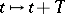. This means that if one replacesby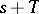, sets, and then replaces the letters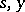by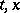, then one should obtain the initial equation. A functional-differential equation is called autonomous if it is invariant under the changefor all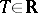.

The order of a functional-differential equation is the order of the highest derivative of the unknown function entering in the equation. So, a functional equation may be regarded as a functional-differential equation of order zero. Hence, the notion of a functional-differential equation generalizes all equations of mathematical analysis for functions of a continuous argument. A similar assertion holds for functions depending on several arguments.

One can naturally define the notions of a functional-differential inequality or inclusion and of a stochastic functional-differential equation, which are similar to the definition of a functional-differential equation.

Consider the following functional-differential equation with finitely many argument deviations: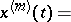(a1)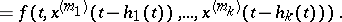Here,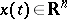, and all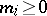,, i.e. all argument deviations are assumed to be non-negative. This property constitutes the definition of a functional-differential equation with retardations. In (a1), the functionand the delays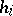are given, andis the unknown function of.

Equation (a1) is called:

a functional-differential equation of retarded type, or a retarded functional-differential equation (abbreviated RDE), if;

a functional-differential equation of neutral type (abbreviated NDE) if;

a functional-differential equation of advanced type (abbreviated ADE) if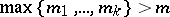. In particular, a retarded functional-differential equation can be characterized as an equation in which the value of the higher derivative for any values of the arguments is defined by the values of the lower derivatives depending on the lesser values of the argument.

Sometimes functional-differential equations with discrete retardations are called equations with time lag, and equations with continuous retardations are called equations with after-effect.

Experience in mathematical modeling has shown that the evolution equations of actual processes with retardations are almost exclusively retarded or neutral functional-differential equations. On the other hand, the investigation of various problems for these equations has revealed that retarded or neutral functional-differential equations have many "nice" mathematical properties (in contrast to functional-differential equations of advanced type).

Equation (a1) can be transformed to a first-order vector equation of higher dimension by taking as new unknown functions the lower derivatives of. Preserving the notationfor the new unknown function andfor the new right-hand side, one can write an RDE as(a2)

and an NDE as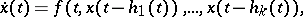(a3)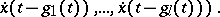Note that any functional-differential equation is equivalent to a hybrid system of ordinary differential equations and functional equations, in particular, difference equations. For example, (a3) is equivalent to the following hybrid system: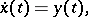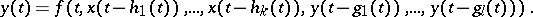More complicated types of retardation have also be considered, and also have real applications. For example, the delay may depend on the unknown solution, and have the form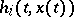. Such delays are sometimes termed auto-regulative.

Similarly, functional-differential equations with retardation and continuous and mixed argument deviations may have the form of an integro-differential or functional integro-differential equation of some structure of Volterra type. The latter means that for anythe integrals depend on the values of the unknown functionon some interval. For example, the continuous analogue of (a2) is the Volterra-type integro-differential equation(a4)Certain classes of equations of the type (a4) can be naturally extended to other functional-differential equations. For example, if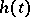takes finite values only, then (a4) has finite after-effect; if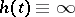, it has infinite after-effect. If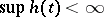, then it has bounded after-effect, and it has unbounded after-effect in the opposite case. Sometimes the after-effect time is interpreted as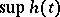; then finiteness, and infiniteness, of after-effect means boundedness, respectively unboundedness, of it.

If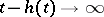as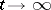, then (a4) is said to have the property of completely forgetting the past. This means that the values of the solutionon any finite-interval do not influence the right-hand side of the equation for sufficiently large. In other words, the rate of change of the state at any moment is determined by the states of the process at preceding moments which are not too remote. Sometimes such equations are said to have fading memory. If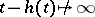as, then either the past is not forgotten (residual phenomena), or is asymptotically forgotten.

Sometimes, Volterra-type integro-differential equations can be reduced to an (in some sense) equivalent system of ordinary differential equations. Delays and equations with after-effect may have a more complicated structure, but they all have the Volterra property: the rate of evolution of the process depends on the past and the present, but not on the future. Keeping this property in mind, one can write the general retarded functional -differential equation in the form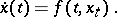(a5)

Here,and(for a given) is the function defined by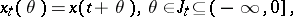whereis a given interval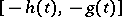or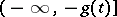. Note that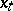may be treated as the fragment of the functionat the left to the point, observed from this point. The right-hand side of (a5) is a function ofand a function of, i.e. to any function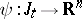in some class of functions there corresponds a vector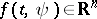.

Equations (a2) and (a4) are particular cases of (a5). Similarly, the general neutral functional-differential equation can be written asVarious problems can be stated for functional-differential equations, e.g. the initial value problem (Cauchy problem), the boundary value problem, the existence of periodic solutions, stability, control, etc. For example, the Cauchy problem (also called the initial problem or basic initial problem) is to find the solution of the equation subjected to a given initial function and initial value. Consider the retarded functional-differential equation (a5) with finite after-effect, and let, for some, the function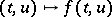be defined for all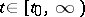,, where. The point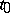is called the initial point for the solution. Assume thatThe initial functionfor (a5) with a prescribed initial pointis given on the initial interval. If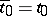then the initial interval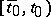is empty and the initial function should not be prescribed. This is a Cauchy problem without previous history. Also the initial value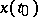of the solution must be given.

It is essential that the solution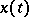must be constructed in the direction of increasing, i.e. on some interval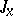with left end. Here,is considered as a prolongation of the initial function, that is,for. Usually, the initial value of the solution is included in the initial function, i.e. the latter is assumed to be prescribed on the interval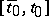and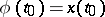.

How to Cite This Entry:
Functional-differential equation. Encyclopedia of Mathematics. URL: http://encyclopediaofmath.org/index.php?title=Functional-differential_equation&oldid=15103
This article was adapted from an original article by V. Kolmanovskii (originator), which appeared in Encyclopedia of Mathematics - ISBN 1402006098. See original article